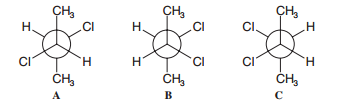Problem: Shown below are Newman projection formulas for (R,R)-, (S,S)-, and (R,S)-2,3-dichlorobutane.  (b) Which formula is a meso compound?

FREE Expert Solution
99% (360 ratings)
Problem Details

Shown below are Newman projection formulas for (R,R)-, (S,S)-, and (R,S)-2,3-dichlorobutane.  (b) Which formula is a meso compound?What scientific concept do you need to know in order to solve this problem?

Our tutors have indicated that to solve this problem you will need to apply the Meso Compound concept. You can view video lessons to learn Meso Compound Or if you need more Meso Compound practice, you can also practice Meso Compound practice problems .

What is the difficulty of this problem?

Our tutors rated the difficulty of Shown below are Newman projection formulas for (R,R)-, (S,S)... as high difficulty.

How long does this problem take to solve?

Our expert Organic tutor, Jonathan took less than a minute to solve this problem. You can follow their steps in the video explanation above.

What professor is this problem relevant for?

Based on our data, we think this problem is relevant for Professor Daoudi's class at UCF.

What textbook is this problem found in?

Our data indicates that this problem or a close variation was asked in . You can also practice practice problems .